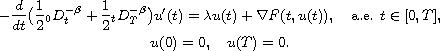Electron. J. Diff. Equ., Vol. 2013 (2013), No. 141, pp. 1-12.

### Existence of solutions to fractional boundary-value problems with a parameter Ya-Ning Li, Hong-Rui Sun, Quan-Guo Zhang

Abstract:
This article concerns the existence of solutions to the fractional boundary-value problemFirst for the eigenvalue problem associated with it, we prove that there is a sequence of positive and increasing real eigenvalues; a characterization of the first eigenvalue is also given. Then under different assumptions on the nonlinearity F(t,u), we show the existence of weak solutions of the problem whenlies in various intervals. Our main tools are variational methods and critical point theorems.

Submitted January 27, 2013. Published June 21, 2013.
Math Subject Classifications: 34A08, 34B09.
Key Words: Fractional differential equation; eigenvalue; critical point theory; boundary value problem.

Show me the PDF file (257 KB), TEX file, and other files for this article.Ya-Ning Li School of Mathematics and Statistics Lanzhou University Lanzhou, Gansu 730000, China email: liyn08@lzu.edu.cn Hong-Rui Sun School of Mathematics and Statistics Lanzhou University Lanzhou, Gansu 730000, China email: hrsun@lzu.edu.cn Quan-Guo Zhang School of Mathematics and Statistics Lanzhou University Lanzhou, Gansu 730000, China email: zhangqg07@lzu.edu.cn